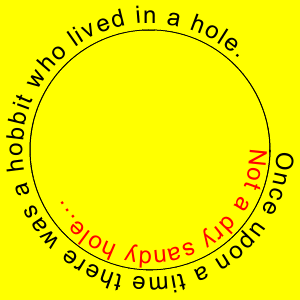DrawShapedText Draw shaped text on the canvas.
 Syntax[C#] ```XRect DrawShapedText(string inText, IEnumerable inPointCoords, DrawOptions inOptions); XRect DrawShapedText(string inText, IEnumerable inPoints, DrawOptions inOptions); ``` [Visual Basic] ```Function DrawShapedText(inText As String, inPointCoords As IEnumerable(Of Double), inOptions As DrawOptions) As XRect Function DrawShapedText(inText As String, inPoints As IEnumerable(Of XPoint), inOptions As DrawOptions) As XRect ```
 ParamsName DescriptioninText The text to draw.inPointCoords The shape point coordinates.inOptions The draw options.inPoints The shape points.return The drawn area.NotesWhen drawing text with this method the text will be aligned along the path specified by the points. You can use Offset and TextVAlign to determine the vertical placement of the text on the path. You can use Kerning and TextHAlign to control the horizontal spacing and placement of characters.

When TextHAlign is less than 0.5 the text is aligned with the first point and drawn left to right. When TextHAlign is 0.5 the middle character of the text is aligned with the middle point. When TextHAlign is bigger than 0.5 the text is aligned with the last point and drawn right to left.

When TextVAlign is less that 0.5 the base of the text is above the points path. When TextVAlign is 0.5 the text is over the path line. When TextVAlign is more than 0.5 the text is below the path line. This can be altered by Offset, which will add a percentage of the line height to the position calculated via TextVAlign.

 See AlsoExampleHere we draw text aligned along a circular path.

[C#]

``````Canvas canvas = new Canvas(300, 300, new XColor(Color.Yellow));
DrawOptions drawOpts = new DrawOptions(canvas);
XPoint[] points = new XPoint;
for (int i = 0; i <= 126; i++) {
double x = (120 * Math.Cos(i / 20.0)) + 150;
double y = (120 * Math.Sin(i / 20.0)) + 150;
points[i] = new XPoint(x, y);
}
drawOpts.VAlign = 0.5;
drawOpts.TextSize = 18;
canvas.DrawShapedText("Once upon a time there was a hobbit who lived in a hole.", points, drawOpts);
drawOpts.Reset(canvas);
drawOpts.Offset = -100;
drawOpts.Kerning = 100;
drawOpts.TextColor = new XColor(Color.Red);
drawOpts.TextSize = 18;
canvas.DrawShapedText("Not a dry sandy hole...", points, drawOpts);
drawOpts.ShapeDrawing = DrawOptions.ShapeDrawingType.Draw;
canvas.DrawPoly(points, drawOpts);
canvas.SaveAs(Server.MapPath("Canvas_DrawShapedText_101.gif"));
```
```

[Visual Basic]

``````Dim canvas As New Canvas(300, 300, New XColor(Color.Yellow))
Dim drawOpts As New DrawOptions(canvas)
Dim points As XPoint() = New XPoint(126) {}
For i As Integer = 0 To 126
Dim x As Double = (120 * Math.Cos(i / 20.0R)) + 150
Dim y As Double = (120 * Math.Sin(i / 20.0R)) + 150
points(i) = New XPoint(x, y)
Next
drawOpts.VAlign = 0.5
drawOpts.TextSize = 18
canvas.DrawShapedText("Once upon a time there was a hobbit who lived in a hole.", points, drawOpts)
drawOpts.Reset(canvas)
drawOpts.Offset = -100
drawOpts.Kerning = 100
drawOpts.TextColor = New XColor(Color.Red)
drawOpts.TextSize = 18
canvas.DrawShapedText("Not a dry sandy hole...", points, drawOpts)
drawOpts.ShapeDrawing = DrawOptions.ShapeDrawingType.Draw
canvas.DrawPoly(points, drawOpts)
canvas.SaveAs(Server.MapPath("Canvas_DrawShapedText_101.gif"))
```
```Canvas_DrawShapedText_101.gif